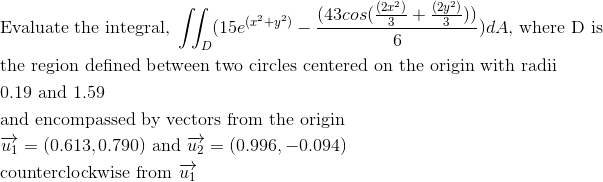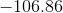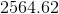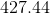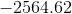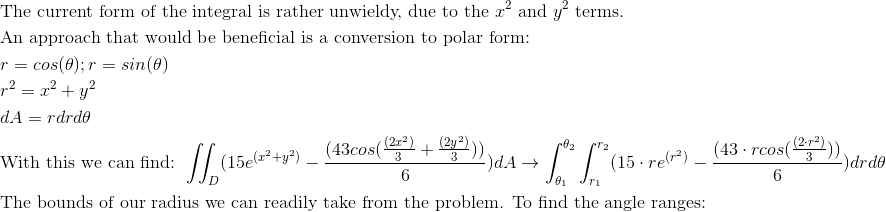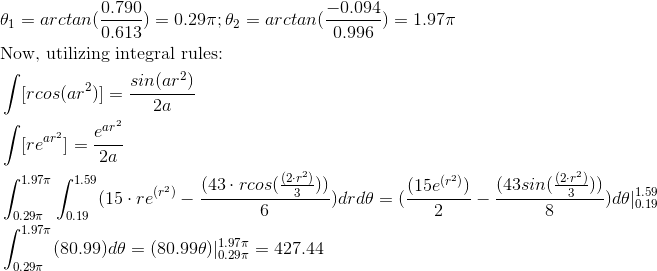Calculus 3 : Double Integrals

Example Questions

Example Question #81 : Double Integration In Polar Coordinates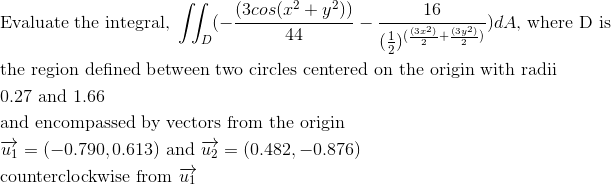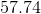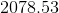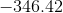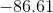Explanation: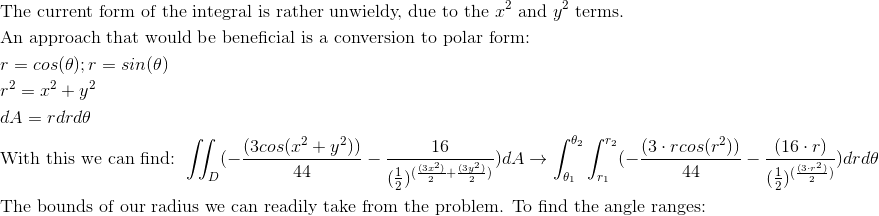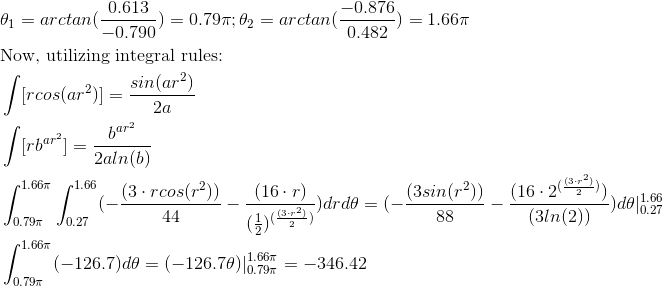Example Question #82 : Double Integration In Polar Coordinates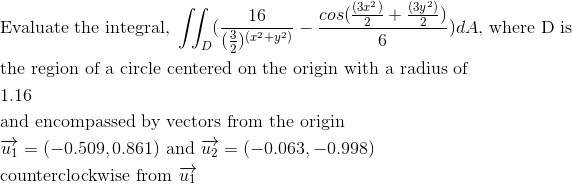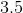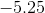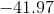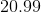Explanation: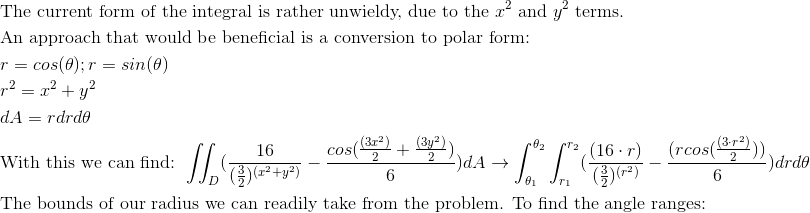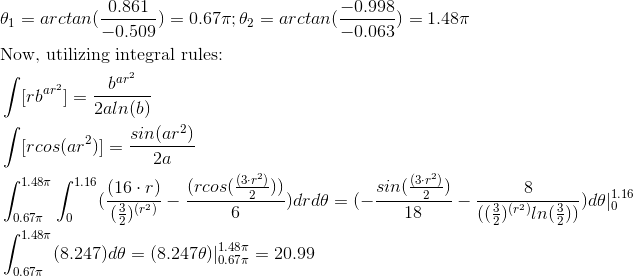Example Question #83 : Double Integration In Polar Coordinates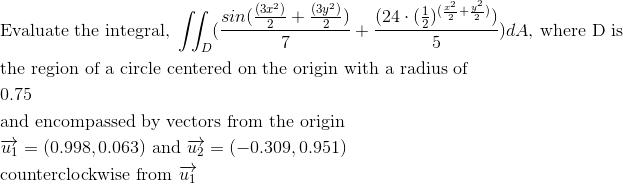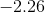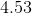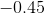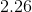Explanation: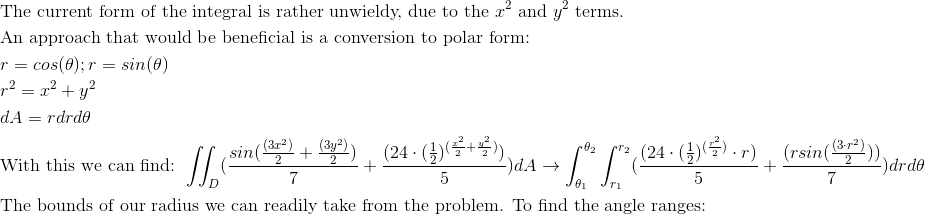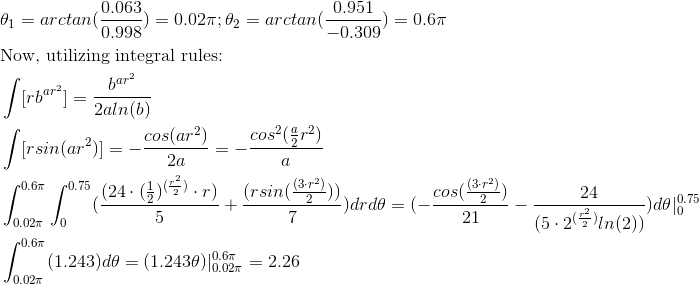Example Question #84 : Double Integration In Polar Coordinates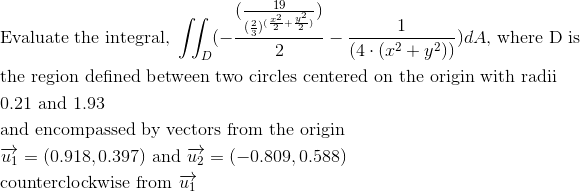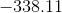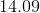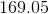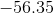Explanation: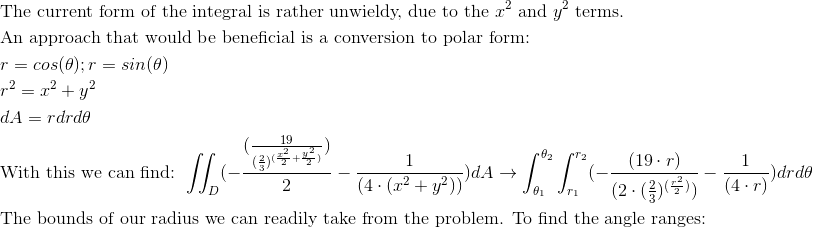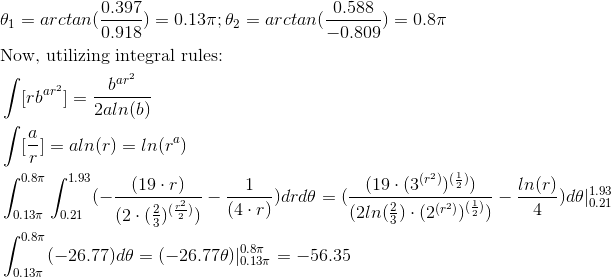Example Question #85 : Double Integration In Polar Coordinates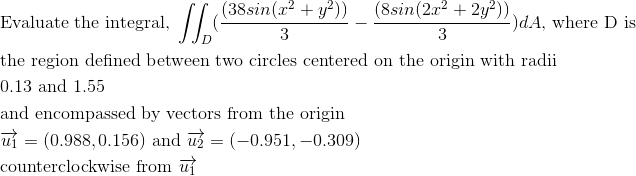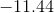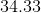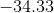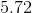Explanation: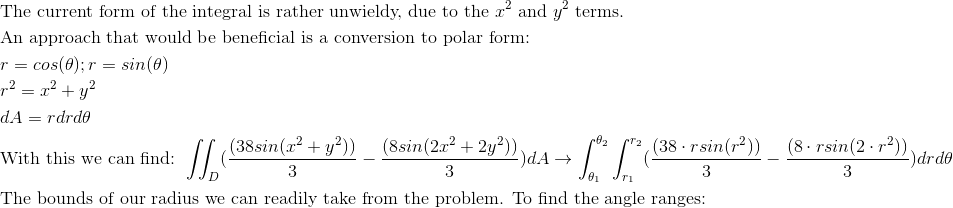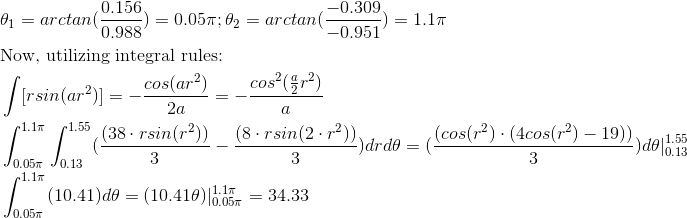Example Question #86 : Double Integration In Polar Coordinates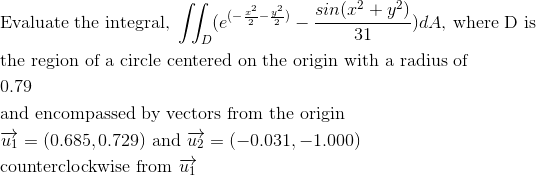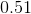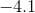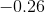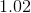Explanation: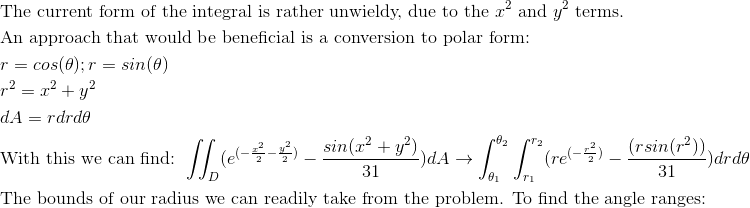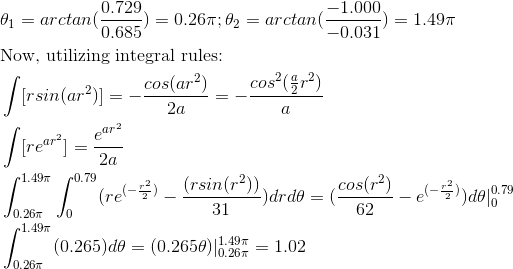Example Question #87 : Double Integration In Polar Coordinates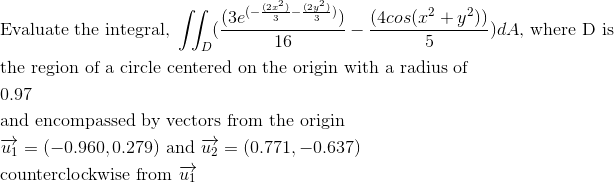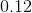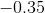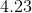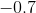Explanation: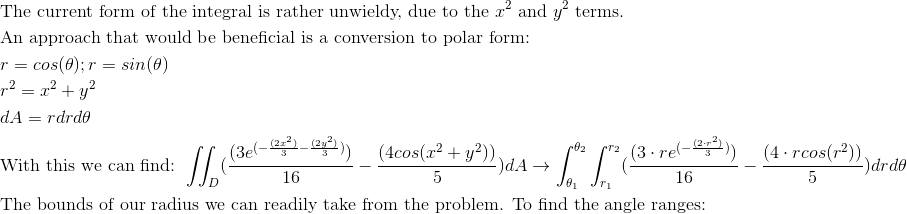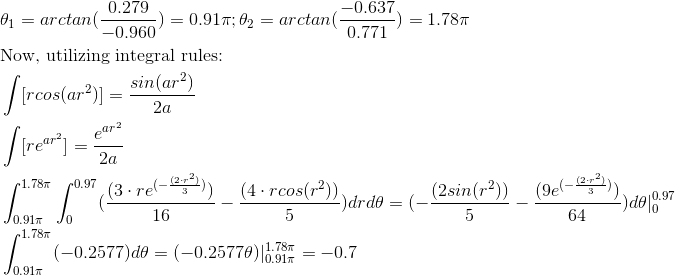Example Question #88 : Double Integration In Polar Coordinates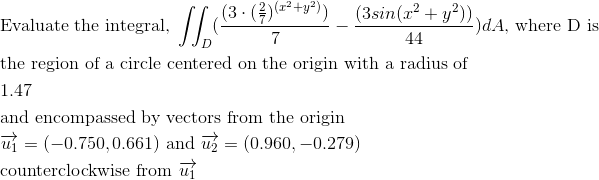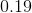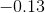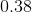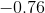Explanation: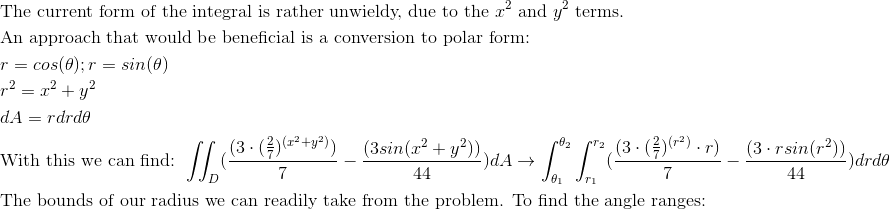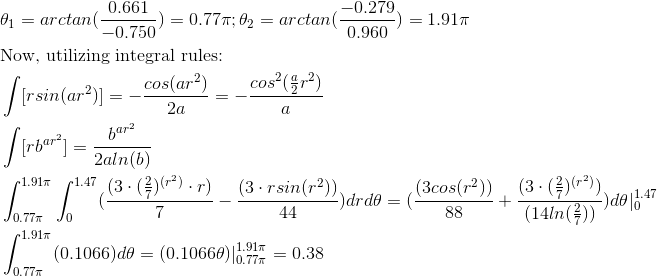Example Question #89 : Double Integration In Polar Coordinates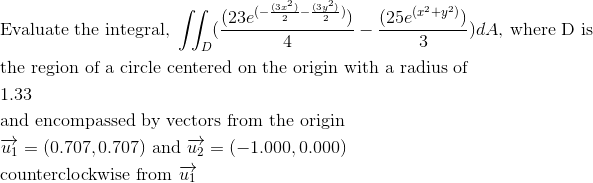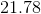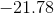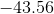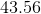Explanation: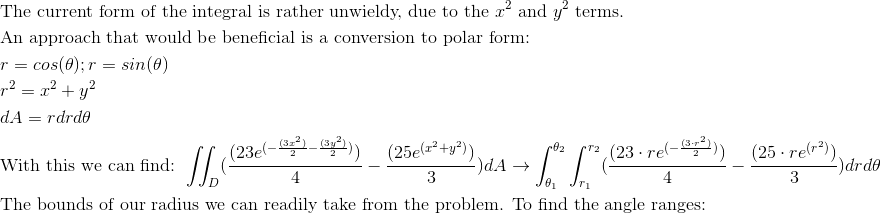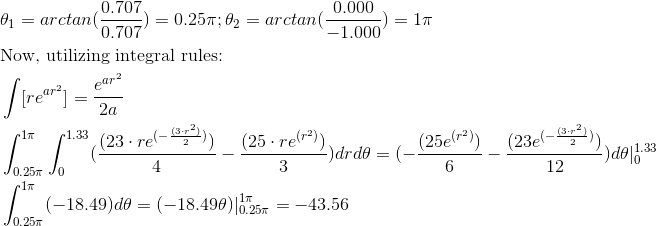Example Question #90 : Double Integration In Polar Coordinates